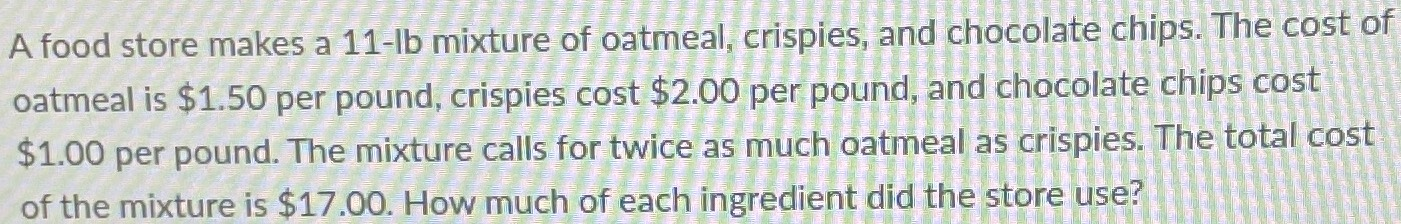### ¿Todavía tienes preguntas de matemáticas?

Pregunte a nuestros tutores expertos
Algebra
PreguntaA food store makes a $$11$$ -lb mixture of oatmeal, crispies, and chocolate chips. The cost of oatmeal is $$\ 1.50$$ per pound, crispies cost $$\ 2.00$$ per pound, and chocolate chips cost $$\ 1.00$$ per pound. The mixture calls for twice as much oatmeal as crispies. The total cost of the mixture is $$\ 17.00$$ . How much of each ingredient did the store use?